## R Project: The Elevation of Tardigrades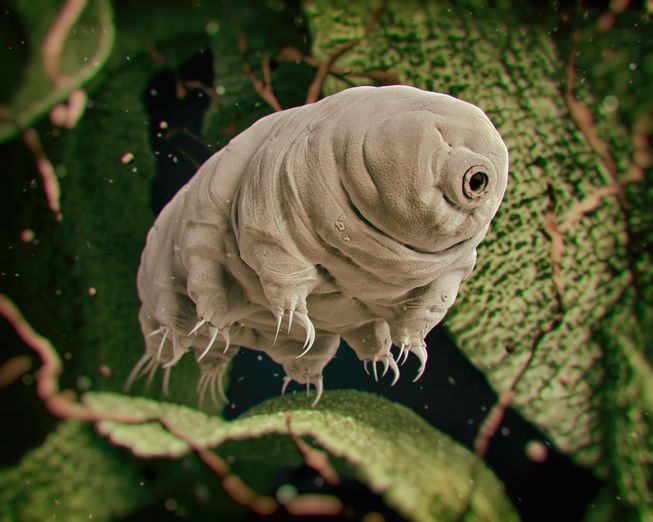Tardigrades (often called "water bears" or "moss piglets") are around 0.5 mm long when fully grown. They are short and plump, with four pairs of legs, each ending in claws (usually four to eight) or sucking disks. They are prevalent in mosses and lichens and feed on plant cells, algae, and small invertebrates.

A recent article on a new species of tardigrades (Macrobiotus shonaicus) first discovered living in a parking lot in Japan claims the mean elevation at which this population of tardigrades can be found is 200 feet above sea-level. (At least, in conducting the associated small-sample means test, with $\sigma$ unknown -- the author found no evidence to the contrary.)

However, in reading the details of her work, you realize that the assumption that the elevations came from a normal population was never checked. The sample, which consisted of only 13 tardigrades of this species, was not of a sufficient size (i.e., $\ge 30$) for the Central Limit Theorem to guarantee that the distribution of sample means was normal for the $t$-test conducted.

One can sometimes look to the sample to get an idea of the shape of the population, but again -- this sample is so small, it is unlikely to provide any statistically significant evidence that the population is not normal. That said, you have an idea...

You reason that one could look at the elevations for other species of tardigrades -- if each of these other species' elevations are distributed in a normal manner, that could at least partially validate the assumption of normality for the $t$-test used in the article.

In a quixotic quest to right a potentially wrongful statistical assumption, you locate two experts on tardigrades, one living in Atlanta, Georgia and another in Denver, Colorado. Each expert studies a different species of tardigrade. Both, you are relieved to discover, are happy to share their data on the locations from which they have harvested various samples of their respective species for their own research. Spending some time with some detailed elevation maps found through a google search, you compile the elevations for each location (including those in Japan) into the following MS Excel spreadsheet:

As previously indicated, you would like to test the claim that both the Georgia and Colorado species' elevations are normally distributed. You know that normal distributions should generally be symmetric and bell-shaped. However, you also know that some other distributions can have these two properties as well (the $t$-distribution comes to mind). You are happy to begin your analysis with checking for skew and visually confirming the sample histograms, but you wish to go beyond these simple checks for normality.

After doing some reading, you settle on two means of testing the normality of data: a QQ-plot and a $\chi^2$ goodness-of-fit-test:

### QQ-Plots

A QQ-plot is a graphical method for comparing two probability distributions by plotting their quantiles against each other. Supposing that one wishes to compare the distribution of $n$ values from a sample against a normal distribution, one first finds a set of cut-off values for the desired quantiles, and then plots these cutoff values against the sorted sample values. As one standard, the cut-off values correspond to those values with the areas under the normal curve and to their left that are shown below.

$$\frac{1}{2n}, \, \frac{3}{2n}, \, \frac{5}{2n}, \, \ldots, \, \frac{2n-1}{2n}$$

If the resulting points approximately follow the line $y=x$, the sample is distributed in a manner approximate to the normal distribution considered.

The following shows two qq-plots where sample data is compared with the normal distribution. The sample associated with the plot on the left is approximately normal, while the one associated with the plot on the right is not.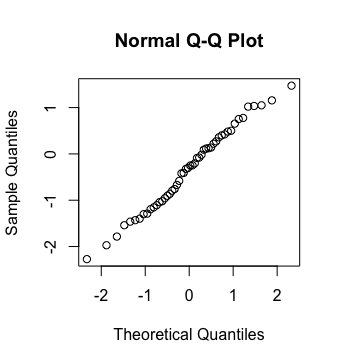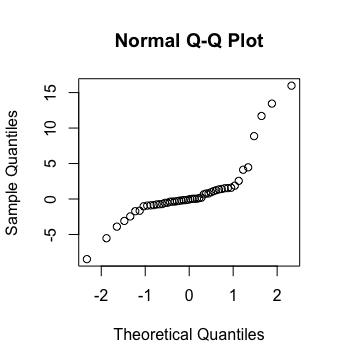an approximately normal sample a non-normal sample

### A $\chi^2$ Goodness-of-Fit Test for Normality

A $\chi^2$ goodness-of-fit test for normality can be constructed in the following way. First, one divides the related normal distribution into intervals that each consist of the same expected proportion, $p$. As an example, the following image shows a standard normal curve divided into quantiles of 20%.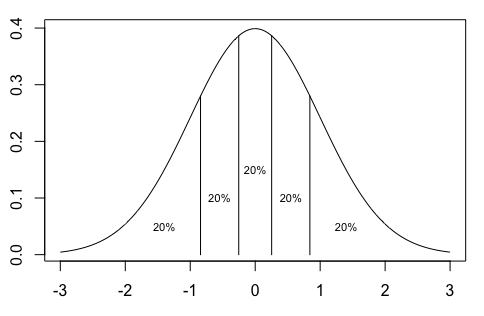Finding the number of elements from the sample (of size $n$) that fall into these respective "bins" gives us the "observed" counts, while the "expected" counts are simply $np$. All that remains is to conduct the appropriate $\chi^2$ goodness-of-fit test on these counts. Rejecting the null hypothesis suggests there is evidence that the distribution is not normal.

### Now, Let's Put All This Together...

With all of the above, in mind, do the following:

1. First, import the excel data into three variables in R: georgia.elevations, colorado.elevations, and japan.elevations. Examine the data in R. You may find that some spaces in the Excel file resulted in the presence of some "NA"'s in your vectors. Note that such things can be removed from a vector d using the following:
d <- d[!is.na(d)]

Do this as needed to the vectors resulting from the import.
2. Write an R function remove.outliers(data) that identifies and prints any outliers (by the IQR test) in data and returns a vector identical to data but with these outliers removed. Use this function to remove any outliers in the Georgia data and any outliers in the Colorado data.

Sample runs:

> ga = remove.outliers(georgia)
 "removed outliers:"
 459 746 745 742
 "removed outliers:"
 5829 6026 5867 7538 7656

3. Then, write an R function skewness.index(data) that behaves similar to the one in the sample runs shown below -- in that it returns Pearson's Skewness Index, $I$, and prints an inference of "no evidence of skew" or "evidence of skew", as appropriate. Use this function to decide if either the Georgia data or the Colorado data is skewed.

Sample runs:

> skewness.index(ga)
 "no evidence of skew"
 "I =  -0.0119948526152501"

> skewness.index(c(1,1,1,1,1,1,1,1,1,1,1,1,1,1,1,1,1,2,2,2,2,2,4,4,5,7,10,20,50))
 "evidence of skew"
 "I =  1.05473803239892"

4. R offers functions qqnorm() and qqplot() that can produce QQ-plots to help decide if sample data follows a normal distribution. However, to ensure that you understand what goes into a QQ-plot, write your own R function my.qqplot(data) that accomplishes the same end and does not rely on the built-in qqnorm() or the related qqplot functions. Additionally, the my.qqplot() function should draw (in red) the line $y=x$ on top of the points plotted to visually assist one in determining if the pattern seen is linear, and show a title and labels on the $x$ and $y$ axes similar to what is shown in the sample output below.

With luck, the Georgia sample will be normally distributed and result in a linear QQ-plot like the one shown in the sample output at left. That said, and just to make sure your function works correctly, try applying it to the dataset given by rchisq(150,2) which is a decidedly non-normal distribution. This should result in a plot that looks more like the sample output at right.

Sample output: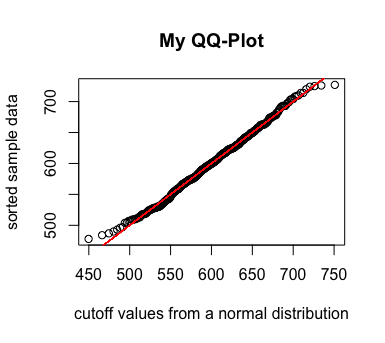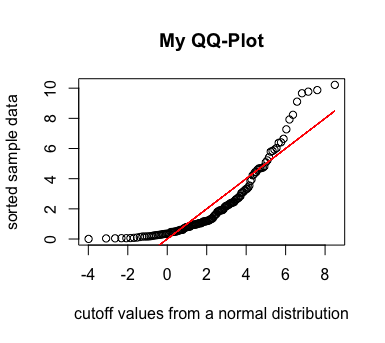5. Then, write an R function norm.fit.test(data,min.exp.in.bin) that uses a normal curve divided up into as many "bins" as possible while still leaving the expected number of elements of data that falls into each of these bins greater than or equal to min.exp.in.bin (you may assume this function is applied to data with at least 10 elements) -- so that the assumptions of the related chi-square goodness-of-fit test are satisfied. Your function should display the observed counts and the expected count for each bin. Then, this function should output the results of this goodness-of-fit test. Use this function with min.exp.in.bin = 20 to test the normality of the georgia and colorado data sets.

Sample output:

> norm.fit.test(co,20)
 "observed:"
 24 15 20 17 25 22 19 25 17 11 25 28 21 13 18 23 23
 "expected count in each bin:"
 20.35294

Chi-squared test for given probabilities

data:  observed
X-squared = 17.387, df = 16, p-value = 0.361

6. Presuming both sets of data are indeed normal, use R to test the hypothesis that the mean elevation for the Japan tardigrades is 200 feet at $\alpha = 0.05$. What inference can be made from your computation? How reliable is this inference? Explain.

7. Excited about your conclusions, you decide to contact one of the two tardigrade experts again -- the one in Denver, Colorado. Upon hearing what you had to say, he responds by saying "I wish you had told me what you were going to use our data for -- I might have saved you some trouble. You see, tardigrades can be found just about everywhere! We just collected ours from the local Denver area. I know for a fact that the guy you contacted down in Georgia obtained his tardegrades from the the local area immediately around his university as well. Both you and the author of that journal article might have a confounding variable to worry about -- Denver is known as the "Mile High City" after all..." What confounding variable might be at play here?

• A vector can be sorted with the sort() function.
• A goodness-of-fit test can be accomplished with the chisq.test(x,p). Notably, x is a vector of observed counts, but p is a vector (of the same length) of proportions (not counts).
• A t test can be accomplished with the t.test() function.